Next: Cohesive Damping Up: Dynamic Cohesive Node/Element Insertion Previous: 2-D Insertion   Contents

## Cohesive Element Stability and System Equilibrium

Thus far, we have only discussed the geometric aspects of the dynamic cohesive element insertion algorithm. It is also important to investigate the effect insertion has on the stability and precision of the dynamic finite element solution. Typical dynamic insertion occurs in regions undergoing dynamic deformations and stresses. In the insertion algorithm described so far, we have not discussed the effect of existing stress state on the insertion method: our basic approach involves simply duplicating all nodal information. The new cohesive element is thus inserted in an ``unstretched'' state, in which the adjacent nodes are superposed.

However, as illustrated below, this ``blind'' insertion may cause oscillations in the response of the cohesive nodes and other approaches have to be adopted. To illustrate this point, let us consider the simple 1-D problem shown in Figure 2.14. It consisting of two segments of equal length with one end fixed at a wall and the other subjected to a prescribed velocity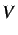. Each segment has a length of, a cross-sectional area of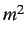, a density of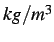, and a Young's modulus of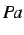. The resulting dilatational wave speed is thus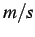, and the critical (Courant) time step is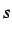. The time step size chosen here is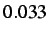due to the inherent instability of the cohesive element to be inserted. A cohesive element is inserted at the center of the system.

For the base solution, we introduce the cohesive element into the mesh at the first time step. Dynamic insertion is investigated by inserting the cohesive element after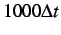(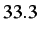) and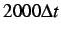(). We run the simulation for a total of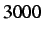time steps (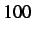) with a constant imposed velocity of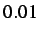.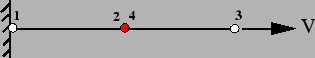To quantify the nodal oscillations resulting from the cohesive element insertion, we plot in Figure 2.15 the evolution of the displacement jump across the cohesive element. As expected, in the reference case for which the cohesive element is present throughout the simulation, the displacement jump increases linearly with time due to the applied velocity boundary condition. No oscillations are observed in that case. On the other hand, insertions after the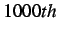and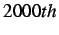time steps create substantial levels of oscillations in the cohesive element response. While the average displacement jump value remains the same as in the reference case, the amplitude of the oscillations increase with the stress level at which the insertion was performed.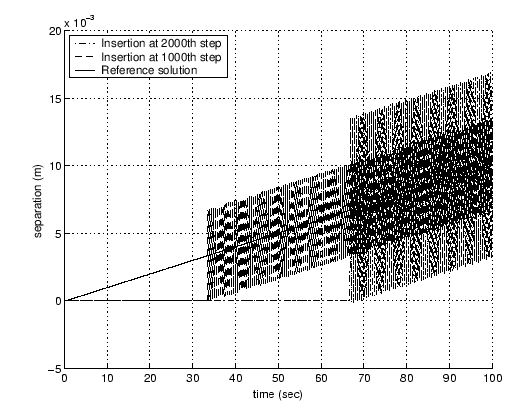These cohesive node oscillations are likewise present in 2-D systems and in order to obtain satisfactory solutions we require that these oscillations be damped out.

SubsectionsNext: Cohesive Damping Up: Dynamic Cohesive Node/Element Insertion Previous: 2-D Insertion   Contents
Mariusz Zaczek 2002-10-13